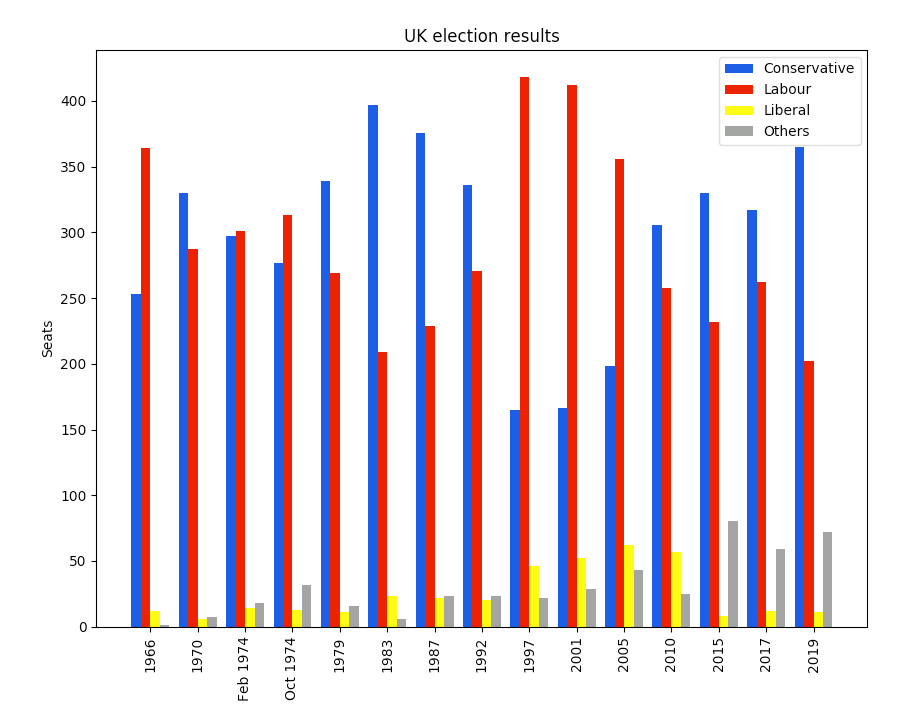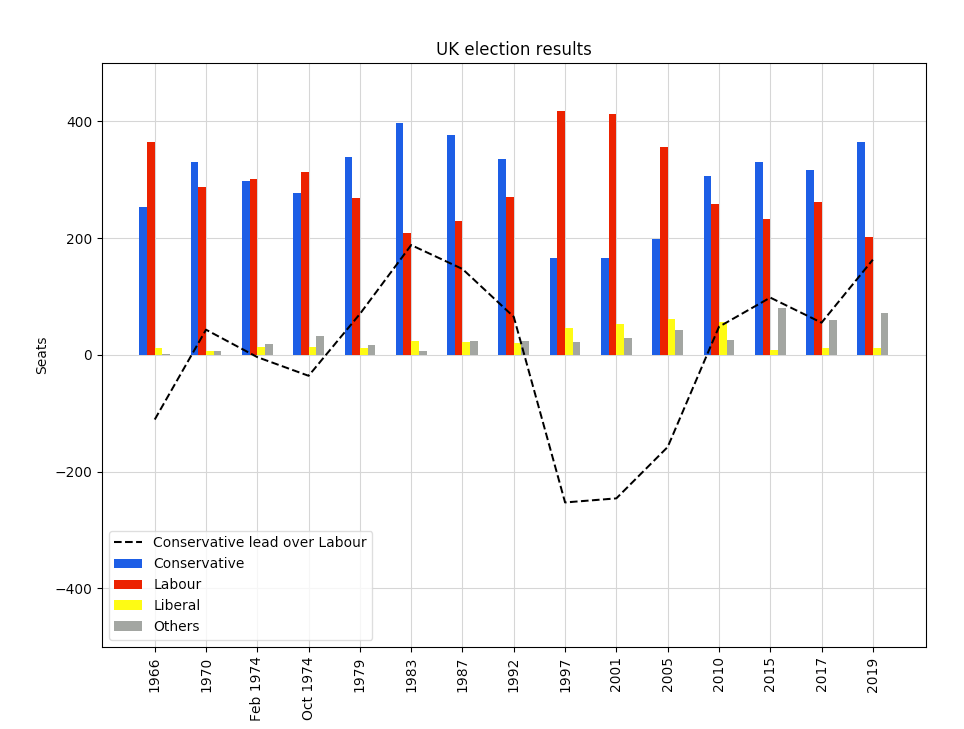# Precision data plotting in Python with Matplotlib

## Matplotlib is the powerhouse of data visualization for Python developers. Here's how to get started.Image by :
Opensource.com

## Subscribe now

Get the highlights in your inbox every week.

Python offers a rich set of options for visualizing data. I'll show you the basics of plotting in Matplotlib by creating a bar chart with grouped bars. It shows election results for the UK between 1966 and 2020:

## britishelectionresults1.pngFor a full comparison of Python plotting libraries, see The 7 most popular ways to plot data in Python.

## Precise and powerful

Matplotlib is the alligator of the plotting zoo. It's been around for a while, but it's still got plenty of bite. Matplotlib gives you precise control over your plots—but, like anything precise and powerful, this sometimes forces you to think harder than you might want to.

To see what I mean, let's start creating the multi-bar plot. Before we go further, note that you may need to tune your Python environment to get this code to run, including the following.

• Running a recent version of Python (instructions for LinuxMac, and Windows)
• Verify you're running a version of Python that works with these libraries

The data is available online and can be imported using pandas:

```import pandas as pd

Now we're ready to go. Start by importing Matplotlib and Numpy:

```import matplotlib.pyplot as plt
import numpy as np```

It's in wide form, meaning there's a column for each political party:

```        year  conservative  labour  liberal  others
0       1966           253     364       12       1
1       1970           330     287        6       7
2   Feb 1974           297     301       14      18
..       ...           ...     ...      ...     ...
12      2015           330     232        8      80
13      2017           317     262       12      59
14      2019           365     202       11      72```

Next, tell Matplotlib that you're creating a figure with a single axis in it. It gives you a Figure and Axis object. If you have several subplots, you have one Figure and several Axes.

```# Create a Figure with one Axis on it
fig, ax = plt.subplots()```

## Making the bar plots

Now add the bar charts themselves. The multi-bar chart is made by drawing four separate bar charts on the same axes—offset each bar chart by a certain amount, so they appear side-by-side. This means you have to work out how to calculate the offset for each bar chart, and if you wanted to add another political party, you'd have to rethink your calculation.

```# The x-values of the bars.
years = df['year']
x = np.arange(len(years))

# The width of the bars (1 = the whole width of the 'year group')
width = 0.15

# Create the bar charts!
ax.bar(x - 3*width/2, df['conservative'], width, label='Conservative', color='#0343df')
ax.bar(x - width/2, df['labour'], width, label='Labour', color='#e50000')
ax.bar(x + width/2, df['liberal'], width, label='Liberal', color='#ffff14')
ax.bar(x + 3*width/2, df['others'], width, label='Others', color='#929591')```

## Axis labels and legend

That's the plot it created on its own, but you still need to add some axis labels and a legend:

```# Notice that features like labels and titles are added in separate steps
ax.set_ylabel('Seats')
ax.set_title('UK election results')

ax.set_xticks(x)    # This ensures we have one tick per year, otherwise we get fewer
ax.set_xticklabels(years.astype(str).values, rotation='vertical')

ax.legend()```

## Make the magic happen

Finally, invoke the magic word to make the plot appear on the screen:

``plt.show()``

Hey, presto!

## britishelectionresults1.pngIt took a bit of mental effort, but you've got a nice, clean plot.

## Power

You can probably see how this API gives you a lot of power. Let's imagine you want to plot a line showing the difference in seats between the Conservative and Labour parties. While you're at it, add a set of gridlines in the background and set some sensible Y-axis limits:

```    ax.plot(x, df['conservative'] - df['labour'], label='Conservative lead over Labour', color='black', linestyle='dashed')
ax.grid(color='#eeeeee')
ax.set_axisbelow(True)
ax.set_ylim([-500, 500])```

## britishelectionresults_with-line.pngYou can get it exactly how you want it because Matplotlib gives a low-level API that gives precise control of how things look and where they are displayed.

If you prefer to run this elsewhere, you can copy this example as an Anvil app here (Note: Anvil requires registration to use).

## Great chart, but can we make it simpler?

All this power is great, but there must be a handful of plots that people want to make all the time. Why can't somebody wrap Matplotlib in a high-level interface that makes things much simpler? That's been done, and it's called Seaborn. We will look into that next time.

In the meantime, congratulations on your first Matplotlib visualization!